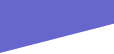Examples of projectsSimulation of work loads (tine forces, roller reactions, etc) with 3D model in Inventor Simulation. These forces and loads can be used in the FE model (below) to calculate stresses, deflections, etc. Unlike hand calculation, which, whilst possible, has to be done repeatedly for different loadings or machine orientations (work, raised, folded, etc), Simulation is dynamic and determines loads and reactions continuously, allowing the peaks to be taken for FE analysis. This, in turn, can illustrate where stress reversals occur, which are a primary source of future fatigue failure in welded joints. Provided the model is complete and the assumptions are realistic (often the area where experience is most required), the loads, weights and forces generated will be representative of the real world.Work loads applied to the component for FE analysis. The forces represent a work situation having peak loads - the 2 tines nearest are loaded to trip, the others to normal work values. The front and rear hitch forces are derived from Simulation at this point. The model is held via the left lower link (A) and these reactions are cross-checked to those calculated in Simulation to ensure the models cross-check correctly. The tines in this analysis are not being evaluated, so are replaced by simple "blocks" of the correct weight and dimensions to simplify the model.FE results for stress: the Inventor model can now be amended and the revised stresses determined until a satisfactory design is achieved. In addition, fatigue life can be determined by comparing different load situations where, for example, stress reversals occur.Images courtesy of Simba International Limited